# High Energy Theory Collaborations Network

Weighted network of collaborations between scientists posting preprints

Weighted network of collaborations between scientists posting preprints on the High-Energy Theory E-Print Archive between Jan 1, 1995 and December 31, 1999. The edge weight indicates the strength of the collaboration between pairs of individuals.

## Examples

### Basic Examples

Retrieve the graph:

 In:=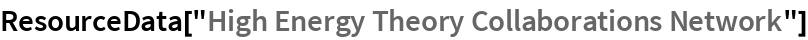Out=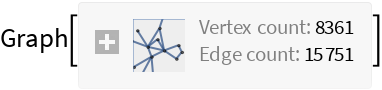Summary properties:

 In:=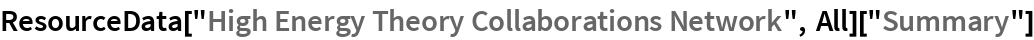Out=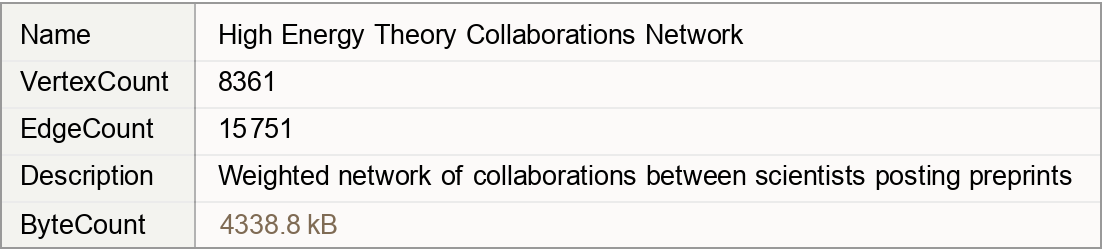### Basic Applications

Pick the author with the most collaborations:

 In:=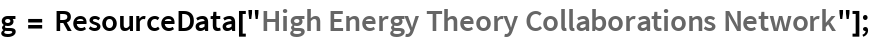In:=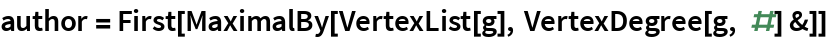Out=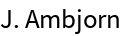Visualize the network around the author with the most collaborations:

 In:=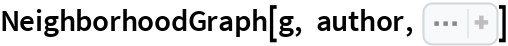Out=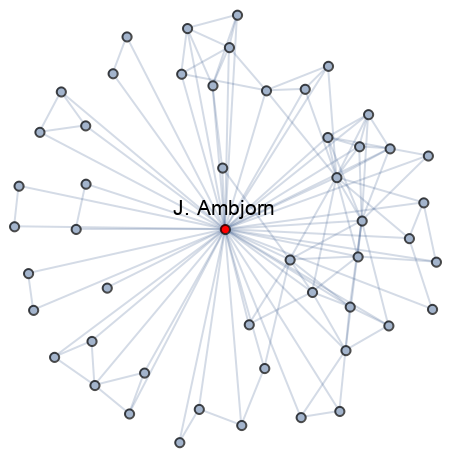Wolfram Research, "High Energy Theory Collaborations Network" from the Wolfram Data Repository (2019)